##### The Japanese Bonsai specialist
Direct order Contact Help / Services Newsletter# Rhododendron gyoten 29040221

› Outdoor bonsai trees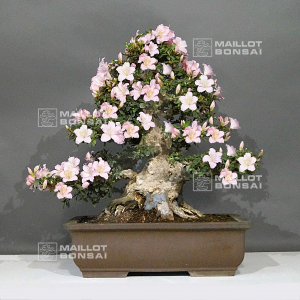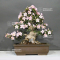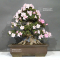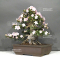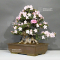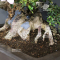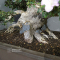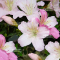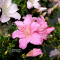ref. : 11681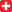Product unavailable

###### Description

Height : 620 mm.

Width : 680 mm.

Pot of : 570*420*170 mm. Nebari of 340/360 mm.

Dimensions of the trunk : 80*170 mm.

Rose goten variety with some clear pink toned flowers and dark pink.

Superb movement of the trunk. It currently has no strong wire marks but some wounds that are healing, covered with mastic. It was caused by shoots on the base of the trunk.

It is currently in a brown colored pot that has a little shard on a side.

This problem can stay without breaking the pot for some years more. It may stay in the same pot, as long as possible because it is a quality pot and, in my opinion, it is in perfect harmony with that rhododenron. These wounds don't clash the whole tree harmony.

To be honest with you, I have to inform you that any bonsaï  has some drawbacks that require to be fixed along years, and when you look at the trees you always find some cutting marks (due to the cut of some big branches that turn too big along years and require to be cut because they are not in harmony with the other branches).

Photographed on the 26 of April 2022.

#some 6.4 #that 6.4 #with 5.4 #harmony 3.7 #trunk 3.5 #years 3.5 #trees 3.5 #rhododendron 3.2 #currently 2.9 #branches 2.8

Formule
(( ROUND((CHAR_LENGTH(b.article_nom)-CHAR_LENGTH(REPLACE(b.article_nom, 'some', '')))/LENGTH('some')) + ROUND((CHAR_LENGTH(b.article_description)-CHAR_LENGTH(REPLACE(b.article_description, 'some', '')))/LENGTH('some')) ) * 6.4) + (( ROUND((CHAR_LENGTH(b.article_nom)-CHAR_LENGTH(REPLACE(b.article_nom, 'that', '')))/LENGTH('that')) + ROUND((CHAR_LENGTH(b.article_description)-CHAR_LENGTH(REPLACE(b.article_description, 'that', '')))/LENGTH('that')) ) * 6.4) + (( ROUND((CHAR_LENGTH(b.article_nom)-CHAR_LENGTH(REPLACE(b.article_nom, 'with', '')))/LENGTH('with')) + ROUND((CHAR_LENGTH(b.article_description)-CHAR_LENGTH(REPLACE(b.article_description, 'with', '')))/LENGTH('with')) ) * 5.4) + (( ROUND((CHAR_LENGTH(b.article_nom)-CHAR_LENGTH(REPLACE(b.article_nom, 'harmony', '')))/LENGTH('harmony')) + ROUND((CHAR_LENGTH(b.article_description)-CHAR_LENGTH(REPLACE(b.article_description, 'harmony', '')))/LENGTH('harmony')) ) * 3.7) + (( ROUND((CHAR_LENGTH(b.article_nom)-CHAR_LENGTH(REPLACE(b.article_nom, 'years', '')))/LENGTH('years')) + ROUND((CHAR_LENGTH(b.article_description)-CHAR_LENGTH(REPLACE(b.article_description, 'years', '')))/LENGTH('years')) ) * 3.5) + (( ROUND((CHAR_LENGTH(b.article_nom)-CHAR_LENGTH(REPLACE(b.article_nom, 'trunk', '')))/LENGTH('trunk')) + ROUND((CHAR_LENGTH(b.article_description)-CHAR_LENGTH(REPLACE(b.article_description, 'trunk', '')))/LENGTH('trunk')) ) * 3.5) + (( ROUND((CHAR_LENGTH(b.article_nom)-CHAR_LENGTH(REPLACE(b.article_nom, 'rhododendron', '')))/LENGTH('rhododendron')) + ROUND((CHAR_LENGTH(b.article_description)-CHAR_LENGTH(REPLACE(b.article_description, 'rhododendron', '')))/LENGTH('rhododendron')) ) * 3.2) + (( ROUND((CHAR_LENGTH(b.article_nom)-CHAR_LENGTH(REPLACE(b.article_nom, 'currently', '')))/LENGTH('currently')) + ROUND((CHAR_LENGTH(b.article_description)-CHAR_LENGTH(REPLACE(b.article_description, 'currently', '')))/LENGTH('currently')) ) * 2.9) + (( ROUND((CHAR_LENGTH(b.article_nom)-CHAR_LENGTH(REPLACE(b.article_nom, 'branches', '')))/LENGTH('branches')) + ROUND((CHAR_LENGTH(b.article_description)-CHAR_LENGTH(REPLACE(b.article_description, 'branches', '')))/LENGTH('branches')) ) * 2.8) + (( ROUND((CHAR_LENGTH(b.article_nom)-CHAR_LENGTH(REPLACE(b.article_nom, 'because', '')))/LENGTH('because')) + ROUND((CHAR_LENGTH(b.article_description)-CHAR_LENGTH(REPLACE(b.article_description, 'because', '')))/LENGTH('because')) ) * 2.7)

## Secure payment## Delivery

Our logistic partners :04 74 55 23 48
Pépinière MAILLOT-BONSAÏ
Le Bois Frazy
01990 RELEVANT - FRANCE
on appointment